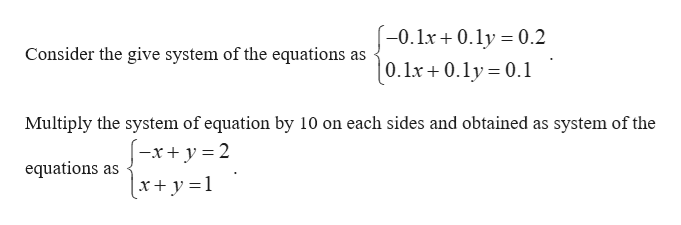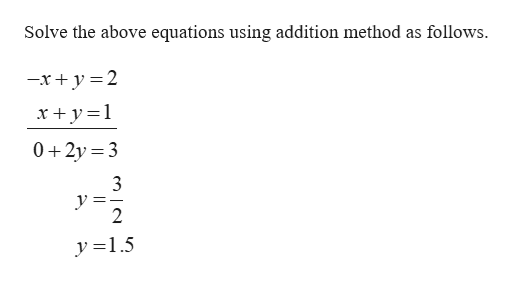# Solve the system of equations by either the addition method or the subtitution method. Express numbers as integers or decimals. -0.1x+0.1y=0.2 0.1x+0.1y=0.1 The solution set is( , )

Question
18 views

Solve the system of equations by either the addition method or the subtitution method. Express numbers as integers or decimals.

-0.1x+0.1y=0.2

0.1x+0.1y=0.1

The solution set is( , )

check_circle

Step 1help_outlineImage Transcriptionclose-0.lx + 0.ly = 0.2 Consider the give system of the equations as |0.1x+ 0.ly = 0.1 Multiply the system of equation by 10 on each sides and obtained as system of the x 2 equations as (x+ y =1 fullscreen
Step 2help_outlineImage TranscriptioncloseSolve the above equations using addition method as follows -x 2 x +y1 0 2y 3 3 V =. 2 y 1.5 fullscreen

### Want to see the full answer?

See Solution

#### Want to see this answer and more?

Solutions are written by subject experts who are available 24/7. Questions are typically answered within 1 hour.*

See Solution
*Response times may vary by subject and question.
Tagged in

### Other